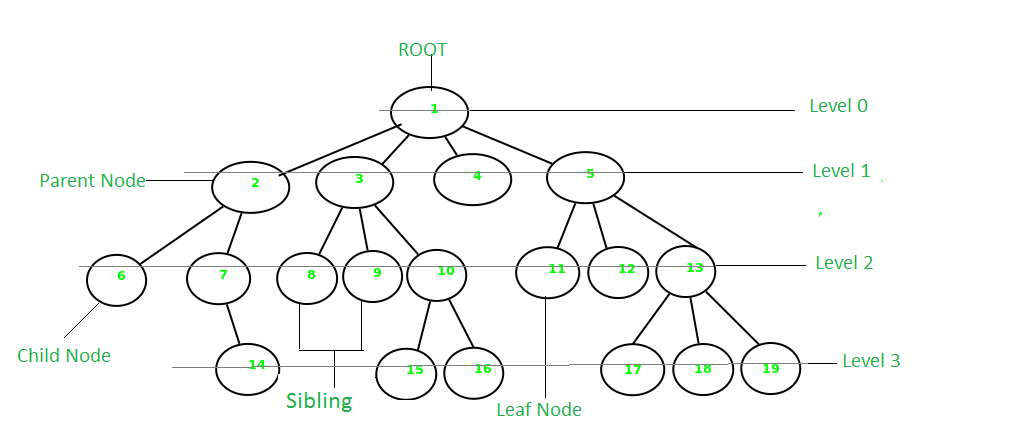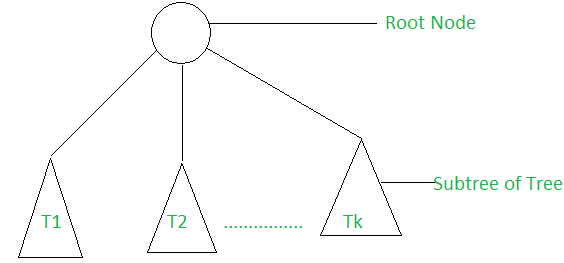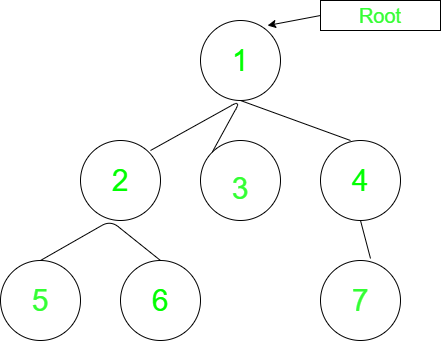Related Articles

# Introduction to Tree Data Structure

• Difficulty Level : Easy
• Last Updated : 02 Sep, 2021

A tree is non-linear and a hierarchical data structure consisting of a collection of nodes such that each node of the tree stores a value, a list of references to nodes (the “children”).Recursive Definition: : A tree consists of a root, and zero or more subtrees T1, T2, … , Tk such that there is an edge from the root of the tree to the root of each subtree.Basic Terminology In Tree Data Structure:

• Parent Node: The node which is a predecessor of a node is called the parent node of that node. {2} is the parent node of {6, 7}.
• Child Node: The node which is the immediate successor of a node is called the child node of that node. Examples: {6, 7} are the child nodes of {2}.
• Root Node: The topmost node of a tree or the node which does not have any parent node is called the root node. {1} is the root node of the tree. A non-empty tree must contain exactly one root node and exactly one path from the root to all other nodes of the tree.
• Degree of a Node: The total count of subtrees attached to that node is called the degree of the node. The degree of a leaf node must be 0. The degree of a tree is the degree of its root. The degree of the node {3} is 3.
• Leaf Node or External Node: The nodes which do not have any child nodes are called leaf nodes. {6, 14, 8, 9, 15, 16, 4, 11, 12, 17, 18, 19} are the leaf nodes of the tree.
• Ancestor of a Node: Any predecessor nodes on the path of the root to that node are called Ancestors of that node. {1, 2} are the parent nodes of the node {7}
• Descendant: Any successor node on the path from the leaf node to that node. {7, 14} are the descendants of the node. {2}.
• Sibling: Children of the same parent node are called siblings. {8, 9, 10} are called siblings.
• Depth of a node: The count of edges from the root to the node. Depth of node {14} is 3.
• Height of a node: The number of edges on the longest path from that node to a leaf. Height of node {3} is 2.
• Height of a tree: The height of a tree is the height of the root node i.e the count of edges from the root to the deepest node. The height of the above tree is 3.
• Level of a node: The count of edges on the path from the root node to that node. The root node has level 0.
• Internal node: A node with at least one child is called Internal Node.
• Neighbour of a Node: Parent or child nodes of that node are called neighbors of that node.
• Subtree: Any node of the tree along with its descendants

Few examples on Tree Data Structure: A code to demonstrate few of the above terminologies has been described below:## C++

 `// C++ program to demonstrate some of the above``// terminologies``#include ``using` `namespace` `std;``// Function to add an edge between vertices x and y``void` `addEdge(``int` `x, ``int` `y, vector >& adj)``{``    ``adj[x].push_back(y);``    ``adj[y].push_back(x);``}``// Function to print the parent of each node``void` `printParents(``int` `node, vector >& adj,``                  ``int` `parent)``{``    ``// current node is Root, thus, has no parent``    ``if` `(parent == 0)``        ``cout << node << ``"->Root"` `<< endl;``    ``else``        ``cout << node << ``"->"` `<< parent << endl;``    ``// Using DFS``    ``for` `(``auto` `cur : adj[node])``        ``if` `(cur != parent)``            ``printParents(cur, adj, node);``}``// Function to print the children of each node``void` `printChildren(``int` `Root, vector >& adj)``{``    ``// Queue for the BFS``    ``queue<``int``> q;``    ``// pushing the root``    ``q.push(Root);``    ``// visit array to keep track of nodes that have been``    ``// visited``    ``int` `vis[adj.size()] = { 0 };``    ``// BFS``    ``while` `(!q.empty()) {``        ``int` `node = q.front();``        ``q.pop();``        ``vis[node] = 1;``        ``cout << node << ``"-> "``;``        ``for` `(``auto` `cur : adj[node])``            ``if` `(vis[cur] == 0) {``                ``cout << cur << ``" "``;``                ``q.push(cur);``            ``}``        ``cout << endl;``    ``}``}``// Function to print the leaf nodes``void` `printLeafNodes(``int` `Root, vector >& adj)``{``    ``// Leaf nodes have only one edge and are not the root``    ``for` `(``int` `i = 1; i < adj.size(); i++)``        ``if` `(adj[i].size() == 1 && i != Root)``            ``cout << i << ``" "``;``    ``cout << endl;``}``// Function to print the degrees of each node``void` `printDegrees(``int` `Root, vector >& adj)``{``    ``for` `(``int` `i = 1; i < adj.size(); i++) {``        ``cout << i << ``": "``;``        ``// Root has noo parent, thus, its degree is equal to``        ``// the edges it is connected to``        ``if` `(i == Root)``            ``cout << adj[i].size() << endl;``        ``else``            ``cout << adj[i].size() - 1 << endl;``    ``}``}``// Driver code``int` `main()``{``    ``// Number of nodes``    ``int` `N = 7, Root = 1;``    ``// Adjacency list to store the tree``    ``vector > adj(N + 1, vector<``int``>());``    ``// Creating the tree``    ``addEdge(1, 2, adj);``    ``addEdge(1, 3, adj);``    ``addEdge(1, 4, adj);``    ``addEdge(2, 5, adj);``    ``addEdge(2, 6, adj);``    ``addEdge(4, 7, adj);``    ``// Printing the parents of each node``    ``cout << ``"The parents of each node are:"` `<< endl;``    ``printParents(Root, adj, 0);` `    ``// Printing the children of each node``    ``cout << ``"The children of each node are:"` `<< endl;``    ``printChildren(Root, adj);` `    ``// Printing the leaf nodes in the tree``    ``cout << ``"The leaf nodes of the tree are:"` `<< endl;``    ``printLeafNodes(Root, adj);` `    ``// Printing the degrees of each node``    ``cout << ``"The degrees of each node are:"` `<< endl;``    ``printDegrees(Root, adj);` `    ``return` `0;``}`

Output
```The parents of each node are:
1->Root
2->1
5->2
6->2
3->1
4->1
7->4
The children of each node are:
1-> 2 3 4
2-> 5 6
3->
4-> 7
5->
6->
7->
The leaf nodes of the tree are:
3 5 6 7
The degrees of each node are:
1: 3
2: 2
3: 0
4: 1
5: 0
6: 0
7: 0```

Attention reader! Don’t stop learning now. Get hold of all the important DSA concepts with the DSA Self Paced Course at a student-friendly price and become industry ready.  To complete your preparation from learning a language to DS Algo and many more,  please refer Complete Interview Preparation Course.

In case you wish to attend live classes with experts, please refer DSA Live Classes for Working Professionals and Competitive Programming Live for Students.

My Personal Notes arrow_drop_up